# Character of a representation of a group

(diff) ← Older revision | Latest revision (diff) | Newer revision → (diff)

In the case of a finite-dimensional representationthis is the functionon the groupdefined by the formulaFor arbitrary continuous representations of a topological groupoverthis definition is generalized as follows: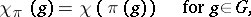whereis a linear functional defined on some idealof the algebragenerated by the family of operators,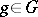, that is invariant under inner automorphisms of. In certain cases the character of a representationis defined as that of the representation of a certain group algebra ofdetermined by(see Character of a representation of an associative algebra).

The character of a direct sum (of a tensor product) of finite-dimensional representations is equal to the sum (the product) of the characters of these representations. The character of a finite-dimensional representation of a group is a function that is constant on classes of conjugate elements; the character of a continuous finite-dimensional unitary representation of a group is a continuous positive-definite function on the group.

In many cases the character of a representation of a group determines the representation uniquely up to equivalence; for example, the character of an irreducible finite-dimensional representation over a field of characteristic 0 determines the representation uniquely up to spatial equivalence; the character of a finite-dimensional continuous unitary representation of a compact group is determining up to unitary equivalence.

The character of a representation of a locally compact groupadmitting an extension to a representation of the algebra of continuous functions of compact support oncan be defined by a measure on; in particular, the character of the regular representation of a unimodular group is given by a probability point measure concentrated at the unit element of. The character of a representationof a Lie groupadmitting an extension to a representation of the algebraof infinitely-differentiable functions of compact support oncan be defined as a generalized function on. Ifis a nilpotent or a linear semi-simple Lie group, then the characters of irreducible unitary representationsofare defined by locally integrable functions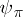according to the formula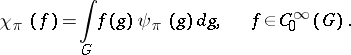These characters determine the representationuniquely up to unitary equivalence.

If the groupis compact, every continuous positive-definite function onthat is constant on classes of conjugate elements can be expanded into a series with respect to the characters of the irreducible representations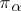of. The series converges uniformly onand the characters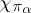form an orthonormal system in the space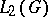that is complete in the subspace of functions inthat are constant on classes of conjugate elements in. If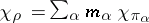is the expansion of the character of a continuous finite-dimensional representationof the groupwith respect to the characters, then the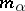are integers, namely, the multiplicities with which theoccur in. Ifis a continuous representation ofin a quasi-complete, barrelled, locally convex topological space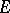, then there exists a maximal subspaceofsuch that the restriction ofto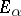is a multiple of, and there is a continuous projection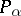ofonto, defined bywhereis the Haar measure onfor which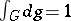.

How to Cite This Entry:
Character of a representation of a group. Encyclopedia of Mathematics. URL: http://encyclopediaofmath.org/index.php?title=Character_of_a_representation_of_a_group&oldid=15563
This article was adapted from an original article by A.I. Shtern (originator), which appeared in Encyclopedia of Mathematics - ISBN 1402006098. See original article